# 06-240/Classnotes For Tuesday December 5

Our remaining goal for this semester is to study the following theorem:

Theorem. Let$A$ be an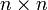$n\times n$ matrix (with entries in some field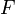$F$) and let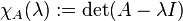$\chi_A(\lambda):=\det(A-\lambda I)$ be the characteristic polynomial of$A$. Assume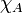$\chi_A$ has$n$ distinct roots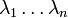$\lambda_1\ldots\lambda_n$, that is,$A$ has$n$ distinct eigenvalues$\lambda_1\ldots\lambda_n$, and let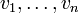$v_1,\ldots,v_n$ be corresponding eigenvectors, so that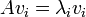$Av_i=\lambda_iv_i$ for all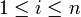$1\leq i\leq n$. Let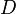$D$ be the diagonal matrix that has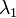$\lambda_1$ through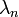$\lambda_n$ on its main diagonal (in order) and let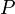$P$ be the matrix whose columns are these eigenvectors: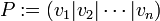$P:=(v_1|v_2|\cdots|v_n)$. Then$P$ is invertible and the following equalities hold:

1.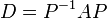$D=P^{-1}AP$ and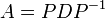$A=PDP^{-1}$.
2. For any positive integer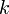$k$ we have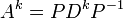$A^k=PD^kP^{-1}$ and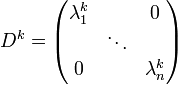$D^k=\begin{pmatrix}\lambda_1^k&&0\\&\ddots&\\0&&\lambda_n^k\end{pmatrix}$.
3. Likewise if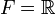$F={\mathbb R}$ and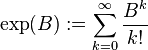$\exp(B):=\sum_{k=0}^\infty\frac{B^k}{k!}$ then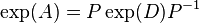$\exp(A)=P\exp(D)P^{-1}$ and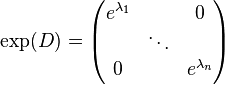$\exp(D)=\begin{pmatrix}e^{\lambda_1}&&0\\&\ddots&\\0&&e^{\lambda_n}\end{pmatrix}$.

Order of the proceedings.

1. Assuming P is invertible, a proof of 1.
2. Proof of 2.
3. Example - the "reproduction of rabbits" matrix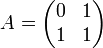$A=\begin{pmatrix}0&1\\1&1\end{pmatrix}$ (see the mathematica session below).
4. Discussion of 3.
5. The relationship with linear transformations and changes of basis.
6.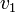$v_1$ thorough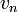$v_n$ form a basis and$P$ is invertible.